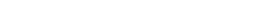How Cheenta works to ensure student success?
Explore the Back-Story

# Test of Mathematics Solution Subjective 37 - The prime 13Test of Mathematics Solution Subjective 37 (from ISI Entrance). The book, Test of Mathematics at 10+2 Level is Published by East West Press. This problem book is indispensable for the preparation of I.S.I. B.Stat and B.Math Entrance.

## Problem

Supposed p is a prime Number such that (p-1)/4 and (p+1)/2 are also primes. Show that p=13.

## Discussion:

p is not 2 or 3 (otherwise (p-1)/4 would not be an integer).Hence p must be an odd prime. Also p-1 is divisible by 4

p = 4t + 1 (say)

(p-1)/4 = t

(p+1)/2 = 2t + 1

Hence t, 2t+1, 4t+1 are all primes.

If t = 3 then these numbers are 3, 7 and 13.

If t is not 3 then t must produce 1 or 2 remainders when divided by 3 (t is a prime, hence cannot be divisible by 3).But 2t +1 is a prime. So it is impossible that 3 divides 2t +1. Hence t cannot be 1 mod 3.

SimilarlyBut 4t +1 is a prime. So it is impossible that 3 divides 4t +1. Hence t cannot be 1 mod 3.

Therefore we can have no other t such that the given condition is satisfied. Hence t must be 3 and the prime must be 13.Test of Mathematics Solution Subjective 37 (from ISI Entrance). The book, Test of Mathematics at 10+2 Level is Published by East West Press. This problem book is indispensable for the preparation of I.S.I. B.Stat and B.Math Entrance.

## Problem

Supposed p is a prime Number such that (p-1)/4 and (p+1)/2 are also primes. Show that p=13.

## Discussion:

p is not 2 or 3 (otherwise (p-1)/4 would not be an integer).Hence p must be an odd prime. Also p-1 is divisible by 4

p = 4t + 1 (say)

(p-1)/4 = t

(p+1)/2 = 2t + 1

Hence t, 2t+1, 4t+1 are all primes.

If t = 3 then these numbers are 3, 7 and 13.

If t is not 3 then t must produce 1 or 2 remainders when divided by 3 (t is a prime, hence cannot be divisible by 3).But 2t +1 is a prime. So it is impossible that 3 divides 2t +1. Hence t cannot be 1 mod 3.

SimilarlyBut 4t +1 is a prime. So it is impossible that 3 divides 4t +1. Hence t cannot be 1 mod 3.

Therefore we can have no other t such that the given condition is satisfied. Hence t must be 3 and the prime must be 13.

### Knowledge Partner